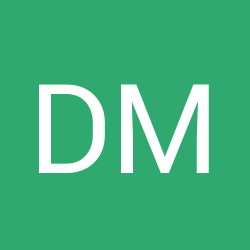# Magic system

•Dennis Müller

Rough exemplary ideas for set theory in first-order logic

 Symbols Magic Ingredients Variables ??? $$\in$$ ??? sets $$\{ x \mid \varphi (x) \}$$ ??? functions ??? relations ??? Quantifiers (binding agents - weak ($$\exists$$) and strong ($$\forall$$)? ) $$\wedge$$ fusing two ingredients $$\to$$ ??? $$\neg$$ anti-ingredient (fusing an ingredient with its anti-ingredient will blow up in your face, unless carefully controlled (proof by contradiction)) Sentences Magic spells ??? Proving Sentences Learning Spells proof steps / techniques gestures / magic words